MORE IN Elements of Civil Engg. & Engg. Mechanics
VTU First Year Engineering (P Cycle) (Semester 1)
Elements of Civil Engg. & Engg. Mechanics
May 2014
Total marks: --
Total time: --
INSTRUCTIONS
(1) Assume appropriate data and state your reasons
(2) Marks are given to the right of every question
(3) Draw neat diagrams wherever necessary

Choose the correct answer for the following :-
1 (a) (i) Geotechnical engineering involves the study of
(A) water
(B) soil
(C) oil
(D) all of these
1 M
1 (a) (ii) A bascule bridge is a
(A) arch bridge
(B) floating bridge
(C) movable bridge
(D) none of these
1 M
1 (a) (iii) Kerbs are the components of
(A) dam
(B) bridge
(D) buildings
1 M
1 (a) (iv) Inspection gallery is a part of
(A) bridge
(B) dam
(C) harbour
(D) airport
1 M
1 (b) Briefly explain the scope of any three fields of civil engineering.
9 M
1 (c) Explain different types of roads.
7 M

Choose the correct answer for the following :-
2 (a) (i) When trying to turn a key into lock, following is applied.
(A) coplanar foces
(B) moment
(C) lever
(D) couple
1 M
2 (a) (ii) The vertical component of a horizontal force is
(A) zero
(B) one
(C) both A and B
(D) two
1 M
2 (a) (iii) Two equal and opposite forces separated by a distance will produce
(A) Translation
(B) rotation
(C) both translation and rotation
(D) none of these
1 M
2 (a) (iv) The resultant of two concurrent forces becomes maximum and minimum, if angle between then is
(A) 0° and 180°
(B) 0° and 90°
(C) 90° and 0°
(D) 0° and 0°
1 M
2 (b) Define force and state its characteristics.
6 M
2 (c) Force acting on the gusset plate of a joint in a bridge truss are shown in Fig. Q2 (c). Determine the values of 'P' and '?' to maintain the equilibrium of the joint.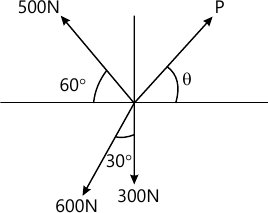10 M

Choose the correct answer for the following :-
3 (a) (i) The process of finding the resultant of a system of forces is called
(A) Resultant
(B) Resolution
(C) Composition
(D) None of these
1 M
3 (a) (ii) If two concurrent forces each of 'P' act at right angles to each other, their resultant is
(A) 2P
(B) P
(C) ?2 P
(D) 2?P
1 M
3 (a) (iii) Conditions of equilibrium for a coplanar concurrent force system is
(A) one
(B) two
(C) three
(D) four
1 M
3 (a) (iv) If two forces are parallel, then they cannot be
(A) Coplanar
(B) Concurrent
(C) Non coplanar
(D) Non concurrent
1 M
3 (b) Two forces F1 and F2 act upon a body. If the magnitude of their resultant is equal to that of F1 and direction perpendicular to F1, then the magnitude and direction of forces F2. Take F1=20 N.
6 M
3 (c) State Varignon's theorem of the moments.
3 M
3 (d) Determine the force P, F and T required to keep the frame in equilibrium.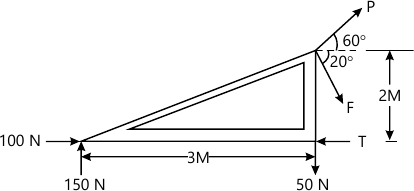7 M

Choose the correct answer for the following :-
4 (a) (i) Centroid of a rectangle of base width 'b' and depth 'd' is
$(A)\ \dfrac {b}{3} \ and \ \dfrac {d}{3}\\(B)\ \dfrac {b}{2} \ and \ \dfrac {d}{2}\\(C)\ \dfrac {b}{4} \ and \ \dfrac {d}{4}\\(D)\ all \ of \ these$
1 M
4 (a) (ii) An axis over which one half of plane figure is just a mirror of the other half axis is
(A) bottom most axis
(B) axis of symmetry
(C) unsymmetrical axis
(D) top most axis
1 M
4 (a) (iii) Centroid conveys some clue about
(A) the orientation of a surface
(B) center of a body
(C) shape and disposition
(D) area of cross section
1 M
4 (a) (iv) The centroid of a semicircle of radius 'r' with respect to its base is
$(A)\ \dfrac {3r}{4\pi}\\(B)\ \dfrac {3r}{8\pi}\\(C)\ \dfrac {4r}{3\pi}\\(D)\ \dfrac {4r}{\pi}$
1 M
4 (b) Determine the centroid of a right angle triangle form first principles.
6 M
4 (c) Find the centroid of the shaded area shown in Fig. Q4(c), obtained by cutting a semicircle of diameter 100 mm from the quadrant of a circle of radius 100 mm.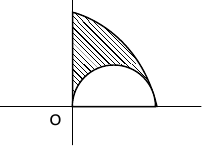10 M

Choose the correct answer for the following :-
5 (a) (i) The force equal and opposite to resultant is called as
(A) resultant
(B) equilibriant
(C) similar force
(D) all of these
1 M
5 (a) (ii) Lami's equation can be applied when number of unknown forces are ______
(A) Two
(B) Five
(C) Four
(D) Three
1 M
5 (a) (iii) In a non concurrent force system, if ?H=0, ?V=0 then the resultant is
(A) zero
(B) horizontal
(C) vertical
(D) moment
1 M
5 (a) (iv) A particle acted upon by two forces of equal magnitude is in equilibrium. The angle between the force is
(A) 0°
(B) 90°
(C) 180°
(D) 45°
1 M
5 (b) State and prove Lami's theorem.
6 M
5 (c) A 100 N sphere is resting in a through as shown in Fig. Q5(c). Find the reactions at the contact points. Assume all contact surfaces are smooth.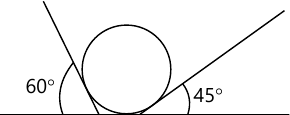6 M
5 (d) An electric lamp fixure weighing 10 N hangs. From a point 'C' by strings Ac at angle 60° and BC at angle 45° as shown in Fig. Q5(d). Determine the forces in strings.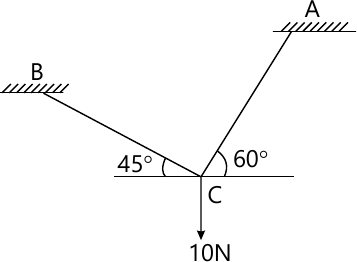4 M

Choose the correct answer for the following :-
6 (a) (i) Support reactions for statically determinate beams can be determined by applying
(A) Varignon's theorem
(B) Lami's theorem
(C) conditions of static equilibrium
(D) none of these
1 M
6 (a) (ii) When loads acts constant rate over given length of beam, it is called as
(B) UDL
(C) UVL
(D) none of these
1 M
6 (a) (iii) A fixed support can have _____ reactions.
(A) 1
(B) 2
(C) 3
(D) 4
1 M
6 (a) (iv) The number of reaction components at an hinged end of a beam is
(A) 0
(B) 2
(C) 3
(D) 1
1 M
6 (b) Find the reactions for a cantilever beam shown in Fig. Q6(b)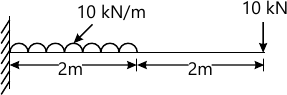6 M
6 (c) Determine the forces in all the members by the method of joints.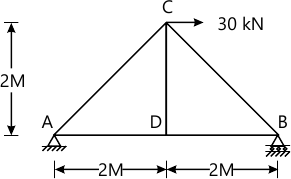10 M

Choose the correct answer for the following :-
7 (a) (i) A friction force always acts ______ to the contact surface.
(A) normal
(B) parallel
(C) at 45°
(D) both A and C
1 M
7 (a) (ii) ______ friction is observed in the flow of liquids and gases.
(A) fluid
(B) static
(C) sliding
(D) kinetic
1 M
7 (a) (iii) Compared to static friction, kinetic friction is
(A) Greater
(B) Smaller
(C) Very large
(D) Zero
1 M
7 (a) (iv) Angle of friction is _____ angle of repose.
(A) =
>
(C) <
(D) both A and B
1 M
7 (b) A block weighing 800N rests on an inclined plane at 12° to the horizontal. If the coefficient of friction is 0.4, find the force required to pull the body up the palne, when the line of the force is
(i) parallel to the plane and
(ii) horizontal.
10 M
7 (c) Define :-
(i) angle of friction
(ii) coefficient of friction
(iii) cone of friction
6 M

Choose the correct answer for the following :-
8 (a) (i) The unit of radius of gyration is
(A) mm
(B) mm2
(C) mm3
(D) mm4
1 M
8 (a) (ii) The moment of inertia of a triangle of base 'b' and height 'h' about its base is
$(A)\ \dfrac {bh^3}{36}\\(B)\ \dfrac {bh^4}{36}\\(C)\ \dfrac {b^3h}{12}\\(D)\ \dfrac {bh^3}{12}$
1 M
8 (a) (iii) The moment of inertia of a side 'b' about its centroidal axis is
$(A)\ \dfrac {b^4}{12}\\(B)\ \dfrac {b^4}{8}\\(C)\ \dfrac {b^4}{36}\\(D)\ \dfrac {b^3}{12}$
1 M
8 (a) (iv) The polar moment of intertia of a circular area of diameter 'd' is given by
$(A)\ \dfrac {\pi d^4}{24}\\(B)\ \dfrac {\pi d^4}{64}\\(C)\ \dfrac {\pi d^4}{32}\\(D)\ \dfrac {\pi d^4}{128}$
1 M
8 (b) State and prove parallel axis theorem :
6 M
8 (c) Calculate the polar moment of inertia of the area shaded in Fig. Q8(c).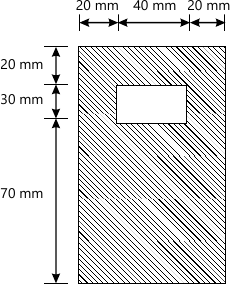10 M

More question papers from Elements of Civil Engg. & Engg. Mechanics